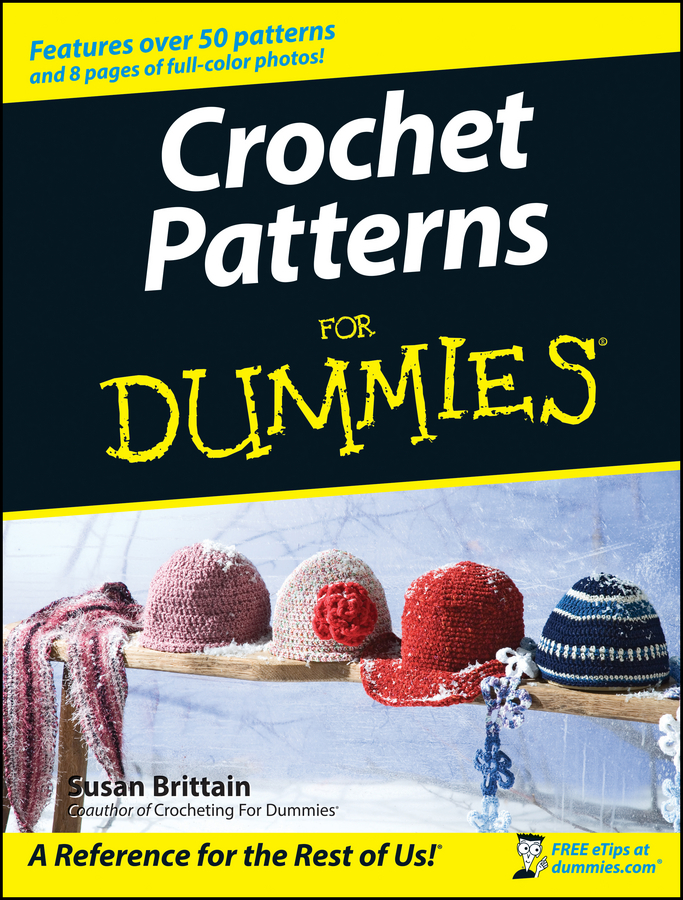##### Crochet Patterns For DummiesReading knitting patterns can be slow work if you're not used to converting from metric measurements to yards, inches, and ounces. Speed up your knitting projects when you're figuring out materials by using these metric conversions. hese are approximations, but they're easy to do in your head and close enough for your knitting needs!

• Yards and meters: A yard is 36 inches. A meter is 39 inches. Multiply yards by 90 percent (0.9) to get meters (100 yards = 90 meters). Multiply meters by 110 percent (1.1) to get yards (100 meters = 110 yards).

• Inches and centimeters: Multiply the number of inches by 2.5 to get centimeters (for example, 4 inches ∞ 2.5 = 10 cm). Divide the number of centimeters by 2.5 to get inches (for example, 10 cm ÷ 2.5 = 4 inches).

• Ounces and grams: 50 grams = 1.75 ounces. 100 grams = 3.5 ounces.